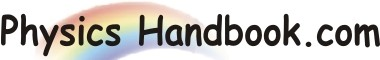HOME TOPICS DEFINITIONS TABLES LAWS INVENTIONS EXPERIMENTS QUIZ VIDEOS
Bode's Law
Bode's law ( also termed as Titius Bode law ) is an equation for representing the approximate distances of the planets from the sun.
The concept was devised and first published in 1766 by Johann D. Titius, a German mathematician and physicist, based on the six planets known at the time: Mercury, Venus, Earth, Mars, Jupiter, and Saturn. The German astronomer Johann E. Bode popularized the law in a book published in 1772, and it became associated with his name.
The law relates the mean distances of the planets from the sun to a simple mathematic progression of numbers. To find the mean distances of the planets, beginning with the following simple sequence of numbers:
0   3   6   12   24   48   96   192   384
With the exception of the first two, the others are simple twice the value of the preceding number.
Adding 4 to each number results,
4   7   10   16   28   52   100   196   388
Then dividing by 10,
0.4   0.7   1.0   1.6   2.8   5.2   10.0   19.6   38.8
The table below shows the formulas and distances for all the planets in the solar system. These distances are measured in astronomical units which equal about 93 million miles (150 million kilometers).
 Body Actual distance (A.U.) Distance by Bode's Law Mercury 0.39 0.4 Venus 0.72 0.7 Earth 1.00 1.0 Mars 1.52 1.6 2.77 2.8 Jupiter 5.20 5.2 Saturn 9.54 10.0 Uranus 19.2 19.6 Neptune 30.06 38.8 Pluto 39.44 77.2